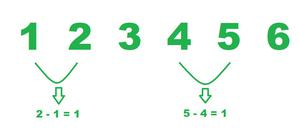Related Articles

# Can an Arithmetic Sequence have a common difference of one?

• Difficulty Level : Basic
• Last Updated : 05 Aug, 2021

The arithmetic definition is – a branch of mathematics that deals usually with the nonnegative real numbers including sometimes the transfinite cardinals and with the application of the operations of addition, subtraction, multiplication, and division to them. The basic operations under arithmetic are addition, subtraction, division and multiplication.

The BODMAS rule is followed to order any operation involving +, −, ×, and ÷. The order of operation is,

B: Brackets

O: Order

D: Division

M: Multiplication

S: Subtraction

### Progression

Progression is a list of numbers (or items) that exhibit a particular pattern. The difference between a sequence and a progression is that to calculate its nth term, a progression has a specific formula i.e. Tn = a + (n-1)d  which is the formula of the nth term of an arithmetic progression.

Arithmetic Progression

An arithmetic progression is a sequence of numbers in which each successive term is the sum of its preceding term and a fixed number. This fixed number or the constant number is called a common difference. In simple terms, it means that the next number in the series is calculated by adding a fixed number to the previous number in the series.

### Common Difference

The difference between each number in an arithmetic sequence or the difference between any two consecutive term. Example: Consider the sequence {1, 5, 9, 13, 17, 21, …} is made by adding 4 each time, therefore it has a “common difference” of 4 (there is a difference of 4 between each number). Suppose, a1, a2, a3, a4 ……………., and is an AP, then; the common difference “d” can be obtained as,

d = a2 – a1 = a3 – a2 = a4 – a3 = ……. = an – an – 1

### Can an Arithmetic Sequence have a common difference of one?

Yes, 1 can be a common difference. A common difference of 1 exists when the next term is just 1 digit more than the previous one. For example, the series of natural numbers, the series of Integers, etc. Lets take a look at this example,

1, 2, 3, 4, 5, 6, 7, 8, 9, 10, 11 … is an AP

which has a common difference between two successive terms (say 1 and 2) equal to 1 which can be obtained (2 -1) = 1.Series of natural numbers with common difference equals to 1 (one) .

Many more examples can be found with a common difference equals to 1.

### Sample Questions

Question 1: Consider the following arithmetic progression :

-3/2, -1/2, 1/2, 3/2…

The common difference can be calculated as (3/2) – (1/2) = 1

Question 2: Consider the following arithmetic progression :

-5, -4 , -3 , -2 , -1 , 0 , 1 , 2 , 3…

The common difference can be calculated as (-3) – (-4) = -3 + 4 = 1

Question 3: Consider the following arithmetic progression :

1.22, 2.22, 3.22, 4.22, 5.22…